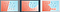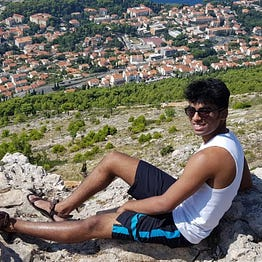# Recap

In the previous posts we showed how connecting several perceptrons together can be a powerful way to find non linear decision boundaries.

But how do we ensure that the decision boundary we find will work well on unseen data?

# Overfitting and underfitting

To elaborate on the question posed above, consider the following models. Which would we consider most likely to succeed on classifying future data points (those that are not currently in our dataset) ?Source: Udacity

We know that the one of the left is too simple, and such a model would be said to ‘under fit’ the data. In the context of MLPs…

# Recap

In the last post we discussed how a perceptron could ‘learn’ the best set of weights and biases to classify some data. We discussed that what ‘best’ really means, in the context of minimising some error function. Finally, we motivated the need to move to a continuous model of the perceptron. Now, we will motivate the need to connect several perceptrons together in order to build more complex non-linear models.

# Motivation

So far, all the models we’ve built using the perceptron have been linear. In reality, a data set is rarely capable of being classified by a simple line. …

# Recap

In the last post, we introduced the concept of a perceptron and how it can be used to model a linear classifier.

A perceptron takes in n input features, x, and multiplies each by a corresponding weight, w, adds on a bias term and finally applies an activation function to the result and spits out a number. Previously we used a step function as the activation in our example of trying to find a model which would classify whether or not a plant would grow, based on time spent in the sun and the amount of water it was given.

# Motivation

The general task of any model is to take in some known quantities and predict some unknown quantity we care about.

Whether it be predicting the price of a house, given its location and the number of bedrooms, or predicting the probability of passing a test, given previous mock test scores. The general idea is the same - take in some things we know, and figure out something we don’t.

So how do we go about building these models? Let’s take the following problem, we want to predict if a plant will grow, given the input features time spent in…## Vishal Jain

Imaging scientist intern